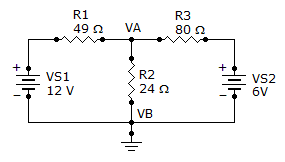# Electrical Engineering - Branch, Loop and Node Analyses - Discussion

Discussion Forum : Branch, Loop and Node Analyses - General Questions (Q.No. 1)
1.
Find the node voltage VA.6 V
12 V
4.25 V
3 V
Explanation:
No answer description is available. Let's discuss.
Discussion:
39 comments Page 1 of 4.

((va-12)/49)+(va/24)+((va-6)/80)=0
((va-12)(1920)+(va)(3920)+(va-6)(1176))/94080=0
va(7016)-30096=0
va=30096/7016
va=4.28

What are node anlayses?

4.25 is not satisfying the nodal equation ?

Will you please tell me the basics formulas related to nodal analysis.

((V-12)/49)+(V/24)+((V-6)/80)=0
or,V=(3762/877)
=4.28 volt
Basic formula of nodal analysis,
For any closed circuit, Summation of the all voltage sources is equals to zero.

But surajit here you have solved taking the summation of current to be zero.

STEPS FOR NODAL ANALYSIS...
STEP1: Choose the nodes and node voltages to be obtained.
STEP2: Choose the currents preferably leaving the node at each
branch connected to each node.
STEP3: Apply Kirchoff's current law at each node with proper sign
convention.
now apply the procedure suggested by our friends.

Steps for Nodal Analysis :.

1. Find the number of Nodes = n.

2. Represent the Nodal voltages = V1, V2, V3, . , Vn.

3. Number of Linear Equations required = Number of nodes = n.

4. Choose any one node as reference node and ground it, so the potential of Reference node = 0.

So one equation is obtained now.

5. To fine the remaining (n-1) equ, Find the voltage sources in the circuit and write equations. If total number of equ are obtained, stop the analysis, otherwise go to the step 6.

6. Find the nodes which have no voltage source and apply KCL. If total number of equ are obtained, stop the analysis otherwise go to step 7.

((NOTE : KCL SHOULD APPLY ONLY AT NON REFERENCE NODES)).

7. Super Node Analysis :.

In this analysis short a voltage source and check step 6. Repeat this step till all the equations are obtained.

((Note : although it's looks like lengthy, but using these steps you can solve any circuit)).

Sunilkumar said:   10 years ago
@Shishir.

According to kcl, at node point, summation of all currents should be zero.

Naveen Pal said:   9 years ago
STEPS FOR NODAL ANALYSIS.

STEP 1: Choose the nodes and node voltages to be obtained.

STEP 2: Choose the currents preferably leaving the node at each
branch connected to each node.

STEP 3: Apply Kirchhoff's current law at each node with proper sign
convention.

Now apply the procedure suggested by our friends.